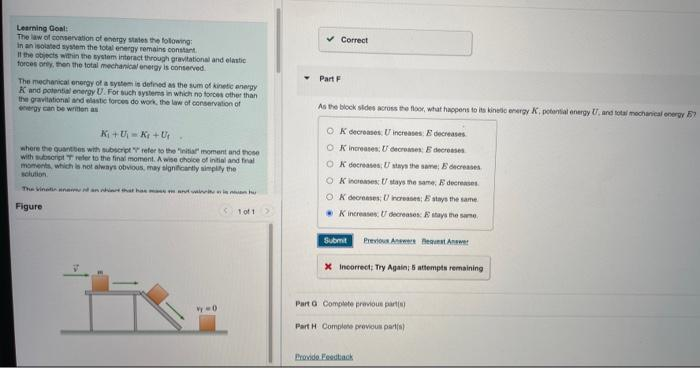# Question Learning Goal: The law of conservation of energy states the following: In an isolated system the total energy remains constant. It the objects within the system interact through gravitational and elastic forces only, then the total mechanical energy is conserved The mechanical energy of a system is defined as the sum of kinetic energy K and potential energy Learning Goal: The law of conservation of energy states the following: In an isolated system the total energy remains constant. It the objects within the system interact through gravitational and elastic forces only, then the total mechanical energy is conserved The mechanical energy of a system is defined as the sum of kinetic energy K and potential energy U. For such systems in which no forces other than the gravitational and elastic forces do work, the law of conservation of energy can be written as K₁+UK+U₁ where the quantities with subscript " refer to the initial moment and those with subscript T refer to the final moment. A wise choice of initial and final moments, which is not always obvious, may significantly simplify the solution. The vinain and an hire that has massaut winning hu 10f1 Figure Vy 0 ✓ Correct Part F As the block sides across the floor, what happens to its kinetic energy K. potential energy U, and total mechanical energy E OK decreases U increases. E decreases OK increases: U decreases E decreases OK decreases; U stays the same; E decreases OK increases: U stays the same, E decreases OK decreases; U increases; Estays the same K increases U decreases: E stays the same Submit Previous Awes Best A X Incorrect; Try Again; 5 attempts remaining Part G Complete previous parts) Part H Complete previous part(s) Provide Feedback